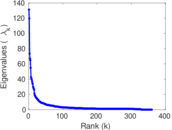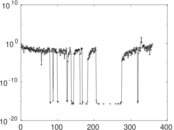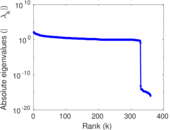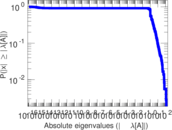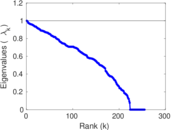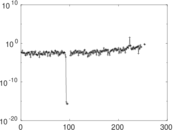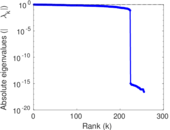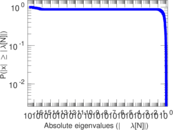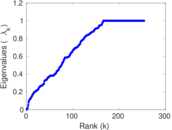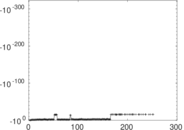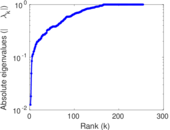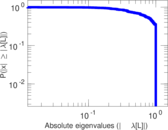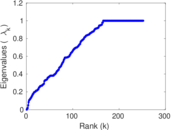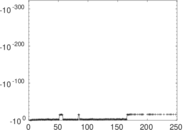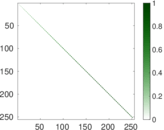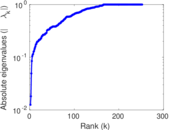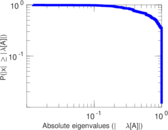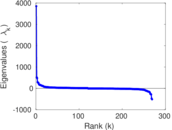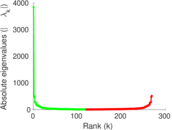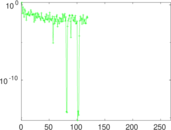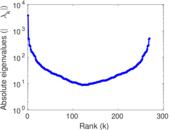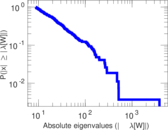# Wikiquote edits (bg)

This is the bipartite edit network of the Bulgarian Wikisource. It contains users and pages from the Bulgarian Wikisource, connected by edit events. Each edge represents an edit. The dataset includes the timestamp of each edit.

 Code `qbg` Internal name `edit-bgwikisource` Name Wikiquote edits (bg) Data source http://dumps.wikimedia.org/ AvailabilityDataset is available for download Consistency checkDataset passed all tests Category Authorship network Dataset timestamp 2017-10-20 Node meaning User, article Edge meaning Edit Network formatBipartite, undirected Edge typeUnweighted, multiple edges Temporal dataEdges are annotated with timestamps

## Statistics

 Size n = 3,862 Left size n1 = 362 Right size n2 = 3,500 Volume m = 9,712 Unique edge count m̿ = 5,071 Wedge count s = 968,866 Claw count z = 239,397,060 Cross count x = 51,363,095,060 Square count q = 27,825 4-Tour count T4 = 4,111,094 Maximum degree dmax = 1,618 Maximum left degree d1max = 1,618 Maximum right degree d2max = 123 Average degree d = 5.029 52 Average left degree d1 = 26.828 7 Average right degree d2 = 2.774 86 Fill p = 0.004 002 37 Average edge multiplicity m̃ = 1.915 20 Size of LCC N = 3,519 Diameter δ = 19 50-Percentile effective diameter δ0.5 = 3.528 01 90-Percentile effective diameter δ0.9 = 5.686 16 Median distance δM = 4 Mean distance δm = 4.231 69 Gini coefficient G = 0.731 104 Balanced inequality ratio P = 0.210 770 Left balanced inequality ratio P1 = 0.113 674 Right balanced inequality ratio P2 = 0.301 483 Relative edge distribution entropy Her = 0.787 745 Power law exponent γ = 4.184 99 Tail power law exponent γt = 2.431 00 Tail power law exponent with p γ3 = 2.431 00 p-value p = 0.000 00 Left tail power law exponent with p γ3,1 = 1.721 00 Left p-value p1 = 0.637 000 Right tail power law exponent with p γ3,2 = 3.971 00 Right p-value p2 = 0.349 000 Degree assortativity ρ = −0.268 787 Degree assortativity p-value pρ = 1.193 09 × 10−84 Spectral norm α = 131.334 Algebraic connectivity a = 0.012 519 8 Spectral separation |λ1[A] / λ2[A]| = 1.098 00 Controllability C = 3,128 Relative controllability Cr = 0.826 202

## Plots

### Fruchterman–Reingold graph drawing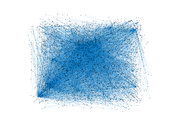### Degree distribution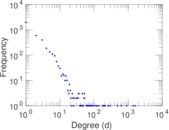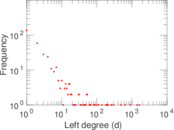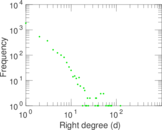### Cumulative degree distribution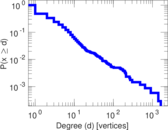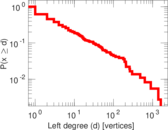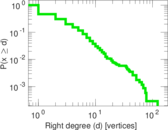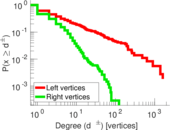### Lorenz curve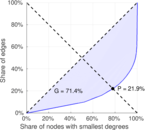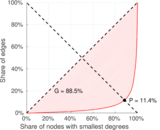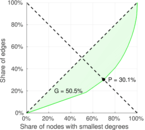### Spectral distribution of the adjacency matrix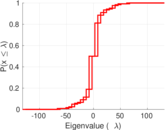### Spectral distribution of the normalized adjacency matrix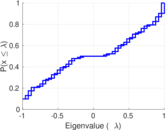### Spectral distribution of the Laplacian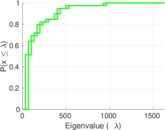### Spectral graph drawing based on the adjacency matrix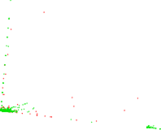### Spectral graph drawing based on the Laplacian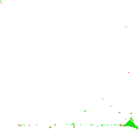### Spectral graph drawing based on the normalized adjacency matrix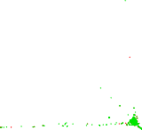### Degree assortativity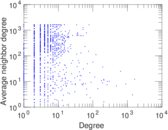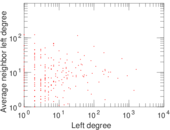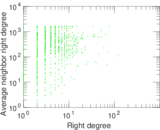### Zipf plot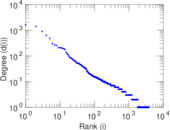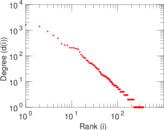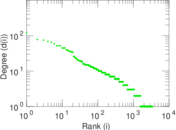### Hop distribution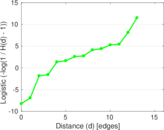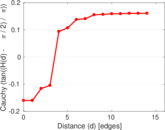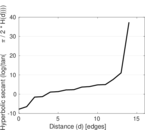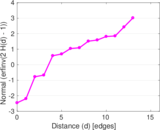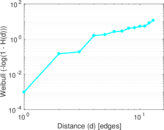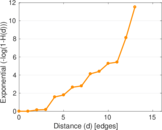### Double Laplacian graph drawing### Delaunay graph drawing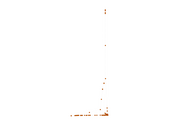### Edge weight/multiplicity distribution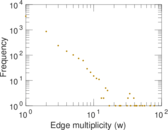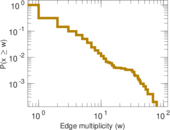### Temporal distribution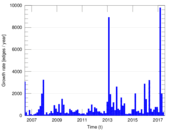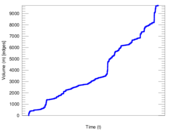### Temporal hop distribution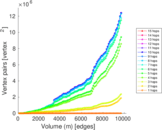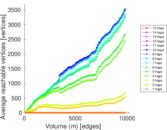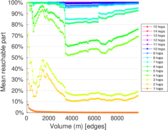### Diameter/density evolution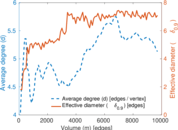### Matrix decompositions plots